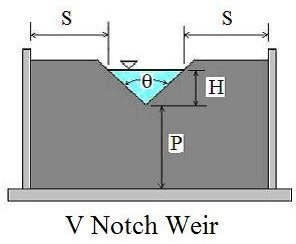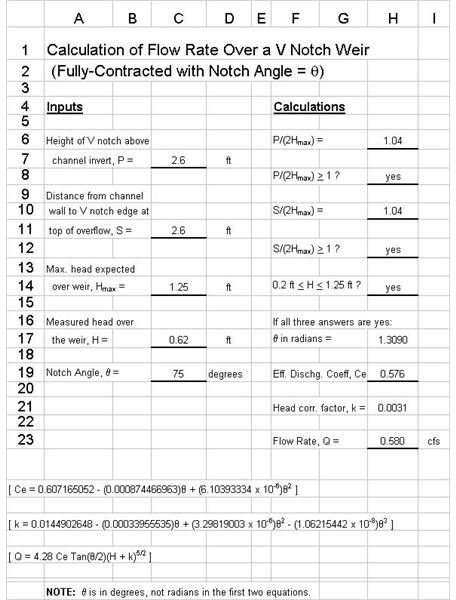# Open Channel Flow Measurement/V Notch Weir Calculations with Excel Spreadsheet Templates

Page content

Calculations for open channel flow measurement with a fully-contracted, 90o V notch weir are pretty straightforward. The use of Excel spreadsheet templates isn’t really required, but does make it easy to check on whether the conditions for fully-contracted flow have been met. If the notch angle is not 90o, then the V-notch weir equation and calculations become somewhat more complicated and the use of Excel spreadsheet formulas can be quite useful. For background information about the V notch weir and the equations for its use in open channel flow rate measurement, see the article, “Open Channel Flow Measurement 4: the V Notch Weir.”

## Excel Spreadsheet Formulas for a Fully-Contracted, 90 Degree, V Notch Weir

The Excel spreadsheet template shown at the left will calculate the flow rate over a fully-contracted, 90o V notch weir, Q, based on given values of the measured head over the weir, H; the height of the V notch vertex above the channel invert, P; the distance from the channel wall to the V notch edge at the top of the overflow; and the maximum expected head over the weir, Hmax.The parameters, H, P, and S are illustrated in the figure of a V notch weir at the right.

The equation, Q = 2.49 H2.48 is used to calculate the flow rate, subject to the conditions that P/(2Hmax) > 1; S/(2Hmax) > 1; and 0.25 < H < 1.25. The Excel spreadsheet formulas are set up to check on whether the three conditions are met and then to calculate the flow rate, Q, if all three conditions are met. The sample spreadsheet shows the checks on the three conditions for given values of P, S, Hmax, and H, and then calculates the water flow rate over the weir. The link below leads to a page from which you can download this spreadsheet template.

## Excel Spreadsheet Formulas for a V Notch Weir with a Notch Angle other than 90 DegreesFor a V notch weir with a notch angle other than 90 degrees, the equation for calculation of the flow rate over the weir is given by the equation: Q = 4.28 Ce Tan(θ/2)(H + k)5/2, where the effective discharge coefficient, Ce, and the head correction factor, k, are both functions of the notch angle, θ. The equations for Ce and k in terms of θ are:

Ce = 0.607165052 - (0.000874466963)θ + (6.10393334 x 10-6)θ2 and

k = 0.0144902648 - (0.00033955535)θ + (3.29819003 x 10-6)θ2 - (1.06215442 x 10-8)θ3

NOTE: The angle, θ, in the above two equations must be in degrees, not radians.

The Excel spreadsheet formulas in the spreadsheet template at the left will calculate Ce, k, and Q for a given head over a V notch weir with a notch angle other than 90o. Values for P, S, and Hmax are needed, because the same conditions given in the previous section must also be met in order to reliably use these equations. This spreadsheet template is similar to the one in the previous section, except it also provides for input of the notch angle, θ, and calculation of Ce and k.

## References and Image Credit

References for further information:

1. Bengtson, Harlan H., Open Channel Flow III - Sharp-Crested Weirs, An online, continuing education course for PDH credit - https://www.online-pdh.com/engcourses/course/view.php?id=87

2. U.S. Dept. of the Interior, Bureau of Reclamation, 2001 revised, 1997 third edition, Water Measurement Manual, available for on-line use or download at: https://www.usbr.gov/pmts/hydraulics_lab/pubs/wmm/index.htm

3. Munson, B. R., Young, D. F., & Okiishi, T. H., Fundamentals of Fluid Mechanics, 4th Ed., New York: John Wiley and Sons, Inc, 2002.

V Notch Weir Image - drawn by H. Bengtson

## This post is part of the series: Excel Spreadsheets for Open Channel Flow Measurement Calculations

These contain Excel spreadsheets that can be downloaded for open channel flow measurement devices, such as V-notch weirs and rectangular weirs. The Excel spreadsheets can be used as a weir flow calculated in each case.